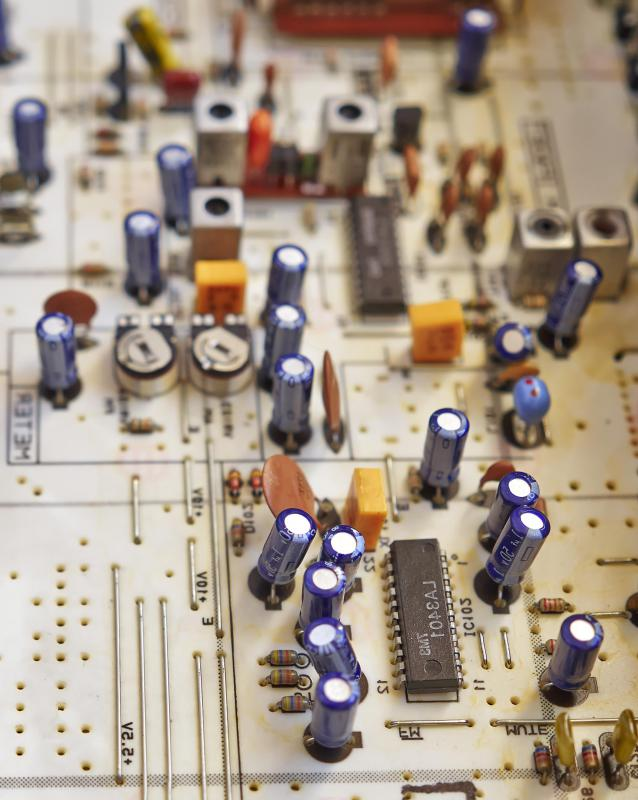# What Is a Bridge Circuit?

Geisha A. Legazpi
Geisha A. LegazpiA resistor is an electronic component that can lower a circuit’s voltage and its flow of electrical current.

A bridge circuit is an electrical circuit that uses a parallel connection of two series legs of components. It usually has four components, with taps at the middle of the parallel legs for comparing output or for special outputs. The bridge circuit may use passive devices like resistors, capacitors, inductors, and even diodes. In the case of diodes, the diode bridge circuit is commonly known as a bridge rectifier or Graetz bridge. The bridge circuit may also use active components such as bipolar and field-effect transistors.

The Wheatstone bridge is a bridge circuit that uses three known resistors and an unknown resistance on the test resistor position. For a direct current (DC) power supply with the negative terminal grounded, the output voltage at the common node of two resistors in series is dependent on the ratio of the resistances in series. If the DC supply voltage is 12 volts (V), with a 6-kilo-ohm (k-ohm) resistance in series with another 6 k-ohm resistance, the output is +6 V referenced to ground. In case another series pair is in parallel with the first set of resistors, and the resistors are the same — for instance, a 3 k-ohm in series with another 3 k-ohm —, the voltage at the node common to both 3 k-ohm resistors is also +6 V referenced to ground, and the resulting voltage across the common nodes is 0 V. The Wheatstone bridge is able to indirectly measure resistance by a voltage null indication, which is a function of the voltage difference between outputs of two voltage dividers.

Specialized versions of the Wheatstone bridge include the Kelvin bridge and the Maxwell bridge. The Kelvin bridge is like a Wheatstone bridge with added resistors that allow it to measure very low resistances, while the Maxwell bridge measures inductance by using a bridge with a capacitance and resistance. In terms of leading and lagging voltages, an inductor in series with a resistor produces a lagging voltage across the resistor. Meanwhile, a resistor in series with a capacitance also produces a lagging voltage across the capacitance. An unknown inductance can be known by detecting for a null voltage across the bridge at a given capacitance.

Bridge-type oscillators that generate sinusoidal waveforms include the bridge T circuit and the Wien bridge oscillator. The bridge T circuit uses a bridge and a T-arrangement of resistance, capacitance, and inductance to control the sinusoidal output for bridge T circuit oscillators and controls output and phase shifts for bridge T circuit filters. Wien bridge oscillators use a resistance-capacitance bridge circuit to control sinusoidal output generated by the oscillator. There are also special bridge circuits like the Murray loop bridge, which is used for estimating locations of faults in cables, and the Fontana bridge, which is a converter from voltage to current making use of operational amplifiers.

## You might also Like## Discuss this Article

Forgot password?
###### Register:
•A resistor is an electronic component that can lower a circuit’s voltage and its flow of electrical current.